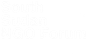# Data Analysis, Modeling and Simulation using Excel Course

(Foscore Development Center) #1

Course Date: 3rd – 7th, June 2019 5 Days

Organizer: Foscore Development Center - www.fdc-k.org

Register here as individual or group to attend :

Introduction

This course is about Statistics and Data Analysis. The course will teach you the advanced concepts related to Statistics and Data Analysis, and help you in applying these concept. Various examples and data-sets will be used and then we will show you how to use Microsoft Excel to perform these calculations.

Course Objective:

• Descriptive and Inferential Statistics.
• Statistical calculations.
• understand statistics from basic to advance using excel

Duration

5 days

Who should attend?

Statistician, analyst, or a budding data scientist and beginners who want to learn how to analyze data with excel.

Course content

• Statistics Introduction and Definitions
• Types of Data in Excel - Text, Numbers, Date/Time, Logical
• Qualitative vs Quantitative Data / Continuous vs Discrete
• Nominal, Ordinal, Interval & Ratio
• Population and Sample (Theory)
• Understanding Data Types
• Understanding Formulas and Functions
• Relative vs Absolute Reference
• Formulas, Functions and Named Ranges
• Descriptive Statistics
• Section Introduction - Descriptive Statistics
• Descriptive Statistics - Central Tendency
• Descriptive Statistics - Variation
• Installing Data Analysis Pack and Calculating Descriptive Statistics
• Descriptive Statistics - Central Tendency
• Descriptive Statistics - Variation (Excel)
• Descriptive Statistics - Shapes
• Descriptive Statistics
• Data Visualization
• Three commonly used Charts
• Create Histogram
• Histogram Shapes
• Create Box and Whisker Plot
• Scatter Diagram
• Create Scatter Plot
• Data Visualization
• Introduction to Basic Probability Concepts
• Basic Probability Concepts
• Calculate Factorial, Permutations and Combinations
• Basic Probability Concepts
• Probability Distributions
• Central Limit Theorem Demonstration
• Normal Probability Distribution
• Binomial Probability Distribution
• Other Distributions Related to Binomial Distribution
• Poisson Distribution
• Probability Distributions
• Introduction Hypothesis Testing
• Hypothesis Testing - Types of Errors
• Hypothesis Testing Explained
• Tests for Mean, Variance and Proportions
• One Sample z Test
• Two Tail Example
• Understanding the p Value
• Introduction to T Functions
• One Sample t Test - Example
• One Sample p Test
• Two Sample z Test
• Two Sample t Test - Equal Variance
• Two Sample t Test - Unequal Variance
• Two Sample t Test - Paired t Test
• Two Sample p Test
• Introduction Tests for Variance (F and Chi Square)
• F Test to Compare Variances
• Plotting F Distribution
• F Distribution Related Functions
• F.TEST Function
• F Test Using Data Analysis Pack
• Chi Square Test for Variance
• Plotting Chi Square Distribution
• Chi Square Distribution Related Functions
• Chi Square Test
• Introduction to ANOVA
• Understanding the concept of ANOVA
• Formulas and calculations in ANOVA
• Performing ANOVA
• Two Factor ANOVA without Replication
• Two Factor ANOVA with Replication
• Goodness of Fit and Contingency Table
• Introduction Goodness of Fit and Contingency Tables
• Goodness of Fit Test
• Contingency Table
• Correlation and Linear Regression
• Introduction to Correlation
• Plotting Scatter Plot and Calculating Correlation Coefficient
• Correlation Coefficient for Multiple Variables
• Correlation Related Functions
• Correlation vs Causation
• Coefficient of Determination
• Manual Calculation
• Slope, Intercept
• Forecast, Linear, Trend & Linest
• Regression using Data Analysis Pack

General Notes

• All our courses can be Tailor-made to participants needs

• The participant must be conversant with English

• Presentations are well guided, practical exercise, web based tutorials and group work. Our facilitators are expert with more than 10 years of experience.

• Upon completion of training the participant will be issued with Foscore Development Center certificate (FDC-K)

• Training will be done at Foscore Development Center (FDC-K) in Nairobi Kenya. We also offer more than five participants training at requested location within Kenya, more than ten participant within east Africa and more than twenty participant all over the world.

• Course duration is flexible and the contents can be modified to fit any number of days.

• The course fee includes facilitation training materials, 2 coffee breaks, buffet lunch and a Certificate of successful completion of Training. Participants will be responsible for their own travel expenses and arrangements, airport transfers, visa application dinners, health/accident insurance and other personal expenses.

• Accommodation, pickup, freight booking and Visa processing arrangement, are done on request, at discounted prices.

• One year free Consultation and Coaching provided after the course.

• Register as a group of more than two and enjoy discount of (10% to 50%) plus free five hour adventure drive to the National Game Park, in Nairobi Kenya.

• Payment should be done two week before commence of the training, to Foscore Development Center account, so as to enable us prepare better for you.

• For any enquiry at: [email protected] or +254712260031

• Website: www.fdc-k.org

• Download our brochure here-

• View course content and register as individual or group using this link-

• View Research and Data Analysis courses calendar 2019

• View All Courses calendar 2019